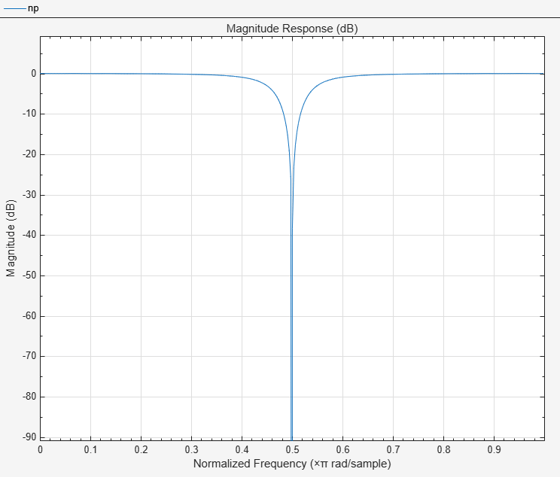# getCenterFrequency

Get center frequency

## Syntax

``CF = getCenterFrequency(npFilter)``

## Description

example

````CF = getCenterFrequency(npFilter)` returns the center frequency of the notch peak filter. If the `Specification` property is set to `'Coefficients'`, the center frequency is determined from the `CenterFrequencyCoefficient` value and the sample rate.```

## Examples

collapse all

Create a `dsp.NotchPeakFilter` object with the `Specification` property set to `'Coefficients'`.

`np = dsp.NotchPeakFilter('Specification','Coefficients')`
```np = dsp.NotchPeakFilter with properties: Specification: 'Coefficients' BandwidthCoefficient: 0.7265 CenterFrequencyCoefficient: 0 SampleRate: 44100 ```

Determine the center frequency of the notch peak filter using the `getCenterFrequency` function. When the `Specification` is set to `'Coefficients'`, the center frequency is determined from the `CenterFrequencyCoefficient` value and the sample rate.

`getCenterFrequency(np)`
```ans = 11025 ```

Visualize the filter response using `fvtool`.

`fvtool(np)`## Input Arguments

collapse all

Notch peak filter whose center frequency is measured, specified as `dsp.NotchPeakFilter` object.

## Output Arguments

collapse all

Center frequency of the filter, returned as a scalar.

Data Types: `double`

### Objects

Introduced in R2014a

## SupportGet trial now